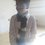# Doubt! Could somebody help me?Alternate text

Hexagon $ABCDEF$ is inscribed in the circle of radius $R$ . $AB=CD=EF=R$. Points $I$, $J$, $K$ are the midpoints of segments $\overline{BC}$, $\overline{DE}$, $\overline{FA}$ respectively. Then prove $\Delta IJK$ is equilateral.

I know this proof is really easy using complex numbers and rotation and stuff, but I'm trying to do this using trigonometry. The method is to find a symmetric expression for one of the sides of $\Delta IJK$ and since it is symmetric, we can claim that the triangle is equilateral.

Note that $\angle IOC = \angle IOB = u$, $\angle JOD = \angle JOE = v$, $\angle KOA = \angle KOF = w$.

I need to find $OI$ and $OJ$. Then using cosine rule, in $\Delta OIJ$ I'll find $IJ$ and then simplify it until it becomes symmetrical in $u$, $v$ and $w$ and $R$. Then we can say that the triangle is equilateral.

I got $OI=R\cos(u)$ and $OJ = R\cos(v)$. Also, in $\Delta OIJ$, $\angle IOJ = 60^{\circ} + u + v$. So using cosine rule, $IJ^{2} = R^{2}\cos^{2}(u) + R^{2}\cos^{2}(v) - 2(R\cos(u))(R\cos(v))(\cos(60^{\circ}+u+v))$ ahead of which I don't know what to do. I need to make this expression symmetrical in $u$, $v$ and $w$ and $R$, of course.

Two helpful points are that $u+v+w=90^{\circ}$ and without loss of generality, we can take the radius of the circle to be one (to simplify calculations). Could someone please finish this for me? Help would be appreciated. Thanks! :)Note by Omkar Kulkarni
6 years, 6 months ago

This discussion board is a place to discuss our Daily Challenges and the math and science related to those challenges. Explanations are more than just a solution — they should explain the steps and thinking strategies that you used to obtain the solution. Comments should further the discussion of math and science.

When posting on Brilliant:

• Use the emojis to react to an explanation, whether you're congratulating a job well done , or just really confused .
• Ask specific questions about the challenge or the steps in somebody's explanation. Well-posed questions can add a lot to the discussion, but posting "I don't understand!" doesn't help anyone.
• Try to contribute something new to the discussion, whether it is an extension, generalization or other idea related to the challenge.

MarkdownAppears as
*italics* or _italics_ italics
**bold** or __bold__ bold
- bulleted- list
• bulleted
• list
1. numbered2. list
1. numbered
2. list
Note: you must add a full line of space before and after lists for them to show up correctly
paragraph 1paragraph 2

paragraph 1

paragraph 2

[example link](https://brilliant.org)example link
> This is a quote
This is a quote
    # I indented these lines
# 4 spaces, and now they show
# up as a code block.

print "hello world"
# I indented these lines
# 4 spaces, and now they show
# up as a code block.

print "hello world"
MathAppears as
Remember to wrap math in $$ ... $$ or $ ... $ to ensure proper formatting.
2 \times 3 $2 \times 3$
2^{34} $2^{34}$
a_{i-1} $a_{i-1}$
\frac{2}{3} $\frac{2}{3}$
\sqrt{2} $\sqrt{2}$
\sum_{i=1}^3 $\sum_{i=1}^3$
\sin \theta $\sin \theta$
\boxed{123} $\boxed{123}$